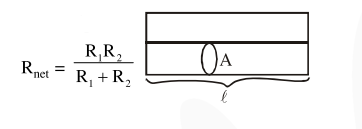# Two wires of same length and thickness having`
Question:

Two wires of same length and thickness having specific resistances $6 \Omega \mathrm{cm}$ and $3 \Omega \mathrm{cm}$ respectively are connected in parallel. The effective resistivity is $\rho \Omega \mathrm{cm}$. The value of $\rho$, to the nearest integer, is_______.

Solution:

$\because$ in parallel$\frac{\rho \ell}{2 \mathrm{~A}}=\frac{\rho_{1} \frac{\ell}{\mathrm{A}} \times \rho_{2} \frac{\ell}{\mathrm{A}}}{\rho_{1} \frac{\ell}{\mathrm{A}}+\rho_{2} \frac{\ell}{\mathrm{A}}}$

$\frac{\rho}{2}=\frac{6 \times 3}{6+3}=2$

$\rho=4$